# Integrals of Some Particular Functions Notes | Study Mathematics (Maths) Class 12 - JEE

## JEE: Integrals of Some Particular Functions Notes | Study Mathematics (Maths) Class 12 - JEE

The document Integrals of Some Particular Functions Notes | Study Mathematics (Maths) Class 12 - JEE is a part of the JEE Course Mathematics (Maths) Class 12.
All you need of JEE at this link: JEE

Introduction

Consider the integral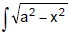dx
If we change the variable from x to θ by the substitution x = a sinθ, then the identity

1 – sin2θ = cos2θ

allows us to get rid of the roots sign because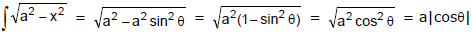Notice the difference between the substitution u = a2 – x2 (in which the new variable is a function of the old one) are the substitution x = a sin θ (the old variable is a function of the new one).

In general, we can make a substitution of the form x = g(t) by using the Substitution Rule in reverse. To make our calculations simpler, we assume that g has an inverse function; that is, g is one-to-one.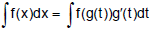This kind of substitution is called inverse substitution.
We can make the inverse substitution x = a sin θ provided that it defines a one-to-one function. This can be accomplished by restricting θ to lie in the interval [–π/2, π/2].

Some Standard Integrals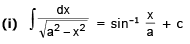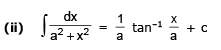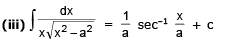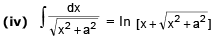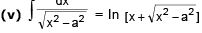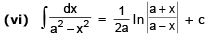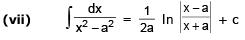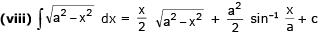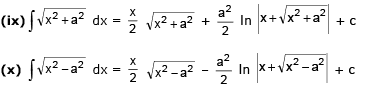Solved Examples

Ex.1 Evaluate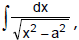where a > 0

Sol.

We let x = a secθ, where 0 < θ < π/2 or π < θ < 3π/2. Then dx = a sec θ tan θ dθ and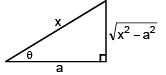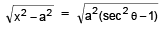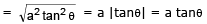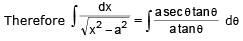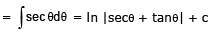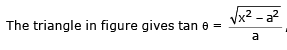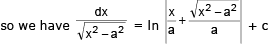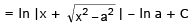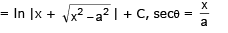Ex.2 Integrate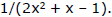Sol.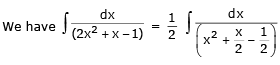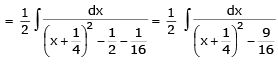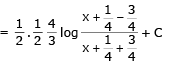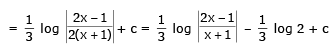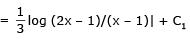Ex.3 Integrate (3x + 1) / (2x2 – 2x + 3).

Sol. Here (d/dx) (2x2 – 2x + 3) = 4x – 2.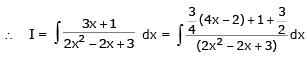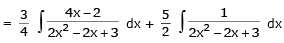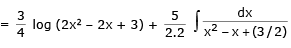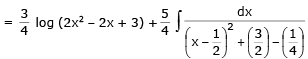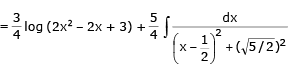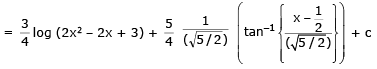Ex.4 Integrate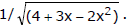Sol.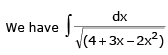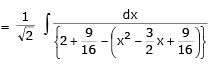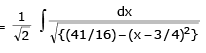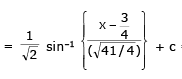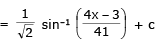Ex.5 Evaluate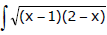dx.

Sol.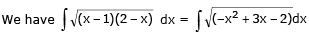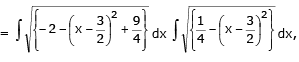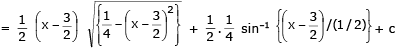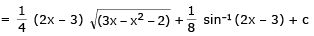Ex.6 Integrate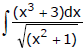Sol.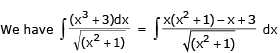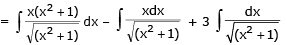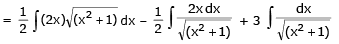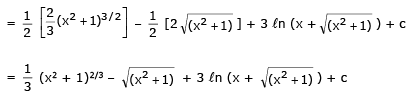Ex.7 Integrate x2/(x4 + x2 + 1)

Sol.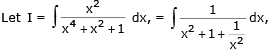dividing the numerator and the denominator both by x2.

Now the denominator  x2 + 1 + 1/x2 can be written either as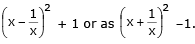The diff. coeff. of  x-1/x is 1 + 1/x2 and that of x + 1/x is 1-1/x2. So we write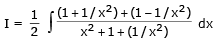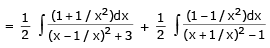In the first integral put x – 1/x = t so that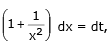and in the second integral put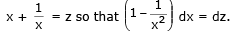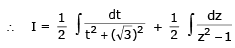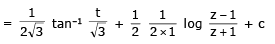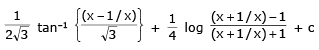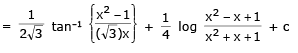Ex.8 Evaluate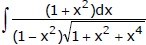Sol.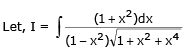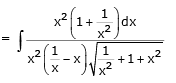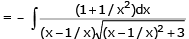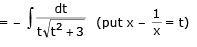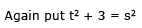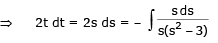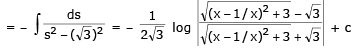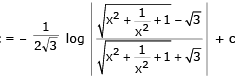Ex.9 Evaluate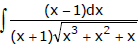Sol.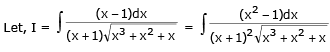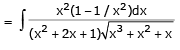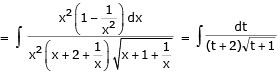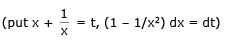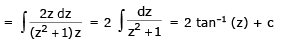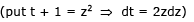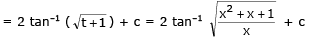Ex.10 Evaluate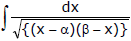Sol.

Put x = αcos2θ + β sin2θ so that dx = 2 (β- α) sinθ cos θ dθ

Also(x – α) = (β - α) sin2θ, and (β - x) = (β - α) cos2θ

Making these substitutions,in the given integral  =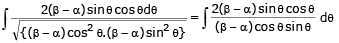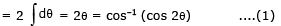But x = αcos2θ + βsin2 θ; 2x = α (1 + cos 2θ) + β(1 - cos 2θ)

i.e., (β - α) cos 2θ = (α + β - 2x) or cos 2θ = (α + β - 2x) / (β - α)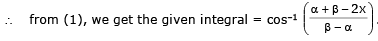Ex.11 Evaluate I =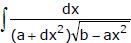Sol.

Substituting ax2 = b sin2 θ ⇒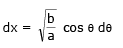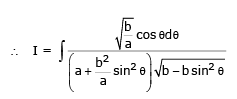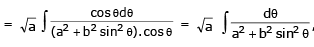dividing Nr and Dr by cos2θ. we get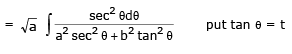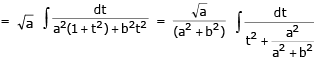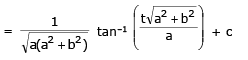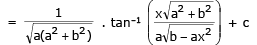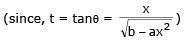Ex.12 Integrate 1/(1 + 3 sin2x).

Sol. Dividing Nr. and Dr. by cos2 x, we have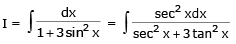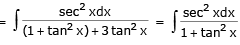Now putting 2 tan x = 5 so that 2 sec2x dx = dt, we have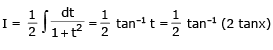The document Integrals of Some Particular Functions Notes | Study Mathematics (Maths) Class 12 - JEE is a part of the JEE Course Mathematics (Maths) Class 12.
All you need of JEE at this link: JEEUse Code STAYHOME200 and get INR 200 additional OFF

## Mathematics (Maths) Class 12

209 videos|209 docs|139 tests

Track your progress, build streaks, highlight & save important lessons and more!

,

,

,

,

,

,

,

,

,

,

,

,

,

,

,

,

,

,

,

,

,

;